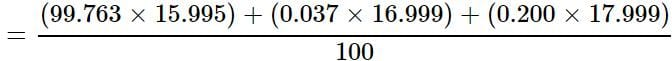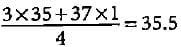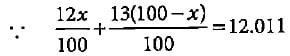# Test: Atomic & Molecular Mass (NCERT)

## 5 Questions MCQ Test Topic-wise MCQ Tests for NEET | Test: Atomic & Molecular Mass (NCERT)

Description
Attempt Test: Atomic & Molecular Mass (NCERT) | 5 questions in 5 minutes | Mock test for NEET preparation | Free important questions MCQ to study Topic-wise MCQ Tests for NEET for NEET Exam | Download free PDF with solutions
QUESTION: 1

### The reference standard used for defining atomic mass is

Solution:

C-12 is used as a standard unit for defining atomic mass unit.

QUESTION: 2

### Atomic masses of elements are usually fractional because

Solution:

Atomic masses are the average atomic masses of the mixture of isotopes and calculated on the basis of relative abundance, hence they are usually fractional.

QUESTION: 3

### Oxygen occurs in nature as a mixture of isotopes 16O, 17O, and 18O having atomic masses of 15.995 u, 16.999 u and 17.999 u and relative abundance of 99.763%, 0.037% and 0.200% respectively. What is the average atomic mass of oxygen?

Solution:

Atomic mass of oxygen= 15.999 u

QUESTION: 4

For every one 37Cl isotope there are three 35Cl isotopes in a sample of chlorine. What will be the ayerage atomic mass of chlorine?

Solution:

Atomic mass of chlorine =QUESTION: 5

Carbon occurs in nature as a mixture of 12C and 13C. The average atomic mass of carbon is 12.011. What is the % abundance of 12C in nature?

Solution:

Let % abundance of  12C = x
% abundance of 13C = 100 - x2x + 1300 - 13x = 1201.1 ⇒ x = 98.9%
Thus, abundance of 12C is 98.9%.Use Code STAYHOME200 and get INR 200 additional OFF Use Coupon Code

### How to Prepare for NEET

Read our guide to prepare for NEET which is created by Toppers & the best Teachers